# Errata

This is where I collect all of my blunders, flaws, fallacies, miscalculations, inaccuracies, and other miscellaneous grammatical and mathematical howlers in Basic Discrete Mathematics. The name of the person who found the erratum is listed at the end. RK is me.

First printing errata

• p. 8: “invitation” should read “invention” (E. Schwab)
• p. 12. Example 1.3.9. “…and the consequent is ‘x is human.'” should read “…and the consequent is ‘x is mortal.'” (RK)
• p. 64. Exercise 2.9.3. The argument is not valid. You can show this is not a valid argument by doing a truth table. (DRE)
• p. 114. Fig 3.37. The set/circle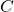should be labeled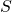. (RK)
• p. 125. “” should read “” (C. Parkes)
• p. 266. Last paragraph.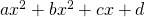should read. (O. Toller)
• p. 285. Exercise 7.1.6. Add “Note that the bus does not traverse a road twice.” (O. Toller)
• p. 329. Last line: “Low-and-behold” should read “Lo-and-behold.” (O. Toller)
• p. 390. Exercise 8.12.14. should read “…one of the candidates given that the committee…”
• p. 391. Line 2: should read “more lively.” (O. Toller)
• p. 422. Example 10.2.1: Add “He fires 4 rounds.” (O. Toller)
• p. 404. Eq. (9.3). Replace Loses with Losses. (RK)
• p. 489. 4th & 5th lines of Proof, 1st term should be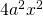, not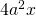. Same issue in 2 places in Exercise B.4.2. (E. Schwab)
• p. 498. Solution to 1.3.6 (d) should be T and not F. (RK & MAE106 2017)
• p. 511. Solution to 1.7.9 (c) is missing. The contrapositive is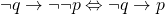. The inverse is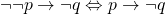. The converse is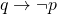. (MS — MAE106 2017)
• p. 526. Solution to 3.3.8. (b) (i) “1, 2, 4, 5, 6” should read “1, 2, 3, 4, 5, 6”. (RK)
• p. 537. Solution 5.2.9. It should read as the following. For the first digit, it can be; that is, there are 9 choices for the first digit. In order for the number to be divisible by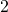or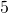, the last digit must be, or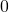. Thus, for the second digit there are 4 choices; that is, you can select from,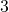,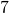, or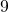. Therefore, there are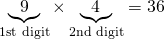numbers that are not divisible byor.

• p. 537. Solution 5.2.10 (b). There is one less choice but there is also the additional choice of 0. If the last digit is a 1, then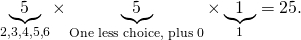If the last digit is a 3, then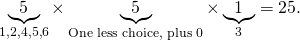If the last digit is a 5, then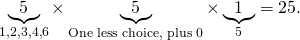By the Sum Principle, there are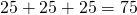ways to do this.

• p. 542. Solution 5.4.12 (b). The solution given in the book overcounts. It should start at 10 instead of 11, since Trent is one of the choices that is not available. The solution should be(RK & C. H. Tsai)

• p. 546. Solution 5.7.10. The solution given in the book overcounts the number of possible hands. Consider cases. Case 1: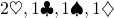which is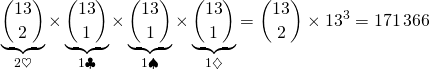.Case 2: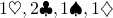which is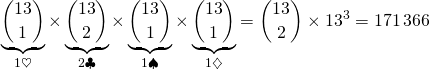.Case 3: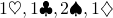which is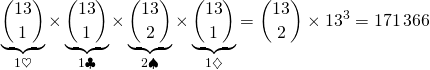.Case 4: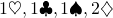which is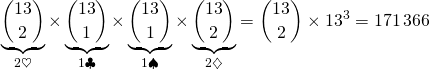.By the Sum Principle, there areways to select the hand as required. (HT — MAE106 2018)
• p. 586. Solution to 6.9.9. The solution given is incorrect since it does not consider the distance traveled by the basketball up and down; the solution is correct for the distance traveled in the downward direction. Replace solution with the following: To help understand the problem, draw a diagram.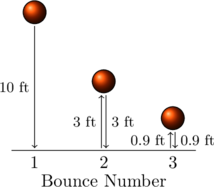The total distance, both up and down, the basketball traveled is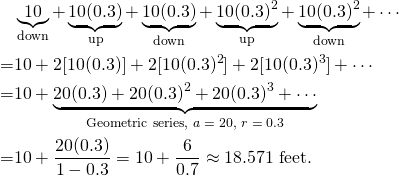(RK)

1.p 266 last para: ax^3 not ax^2
p 285 ex 7.1.6: need to state that you don’t traverse a road twice?
p 329 last line: “LO and behold”?
p 340 8.7.19: problem needs a bit more specification I think
p 391 l 2 “more/livelier”?
p 422 ex 10.2.1: number of shots needs specifying?
p 447 qu 10.3.9: this isn’t a Poisson process! Need a fixed time or number interval (and then it’s probably binomial)
p 632 solution to 8.8.15. Second sentence is wrong I think: it’s “given that the drawer picked from the box contains a gold medal.” In fact put the way you have put it here the answer is pretty obvious and I think the last sentence should be “this makes good sense as” rather than “this seems counter-intuitive”

1.Thanks!

2.p269 ex 6.10.2. summations are indexed using i so expression being summed should use i not n

1.Thank you!

2.p. 489: 4th & 5th lines of Proof, 1st term should be 4a^2x^2, not 4a^2x. Same issue in 2 places in Exercise B.4.2.

3.p. 8: “… truth table, an invitation developed in 1893 by the American logician Charles Sanders Peirce …” “invitation?” “invention?”

1.Yes. It should have been invention.

2.Errata on errata: Solution to 5.4.12 (c) should be …
I think it is 5.4.12.(b) not 5.4.12.(c).

1.Thank you! I’ve changed it.

2.